High School Math : Integrals

Example Questions

1 2 3 4 5 7 Next →

Example Question #71 : Asymptotic And Unbounded Behavior

Determine the indefinite integral: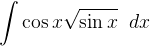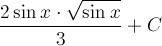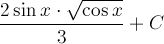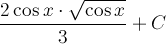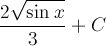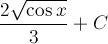Explanation:

Set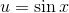. Then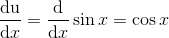.

and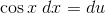The integral becomes: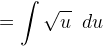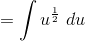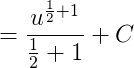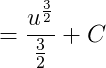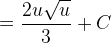Substitute back: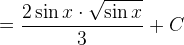Example Question #4 : Finding Integrals By Substitution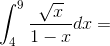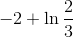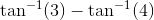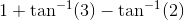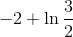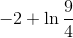Explanation:

This integral will require a u-substitution.

Let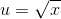.

Then, differentiating both sides,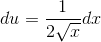.

We need to solve for dx in order to replace all x terms with u terms.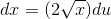.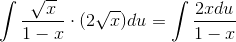This is a little tricky because we stilll have x and u terms mixed together. We need to go back to our original substitution.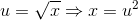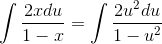Now we have an integral that looks more manageable. First, however, we can't forget about the bounds of the definite integral. We were asked to evaluate the integral fromto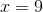. Because, the bounds will change to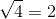and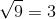.

Essentially, we have made the following transformation: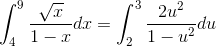.

The latter integral is easier to evaluate.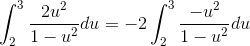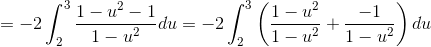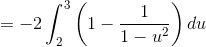At this point, we can separate the integral into two smaller integrals.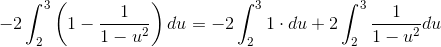.

The integral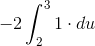evaluates to -2, so now we just need to worry about the other integral. This will require the use of partial fraction decomposition. We need to rewrite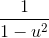as the sum of two fractions.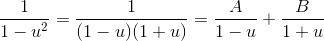We need to solve for the values of A and B.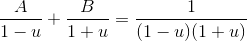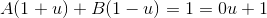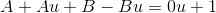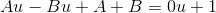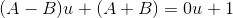This means that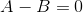and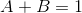. This is a relatively simple system of equations to solve, so I won't go into detail. The end result is that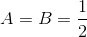.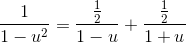Let's now go back to the integral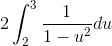.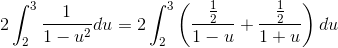Distribute the 2 to both integrals and separate it into two integrals.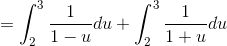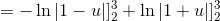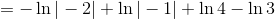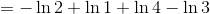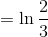.

Remember we need to add this value back to the value of, which we already determined to be -2.

The final answer is.

Example Question #5 : Finding Integrals By Substitution

Evaluate: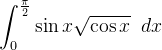Explanation:

Set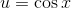.

Then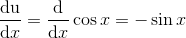and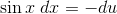.

Also, since, the limits of integration change to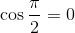and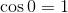.

Substitute: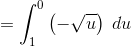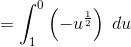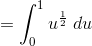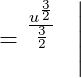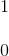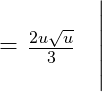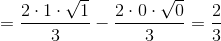1 2 3 4 5 7 Next →